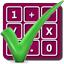#### Arithmetic of Computers

#### from Tenscope Limited

By using this site, you are accepting "session" cookies, as set out in the site's Privacy Policy
Cookies are also used to remember which page of the book you last viewed, so that when you revisit the site you automatically return to the last page you visited.

### Lesson 8

#### Page 259

So far we have discussed converting numbers from octal to decimal, and from decimal to octal. Now it is time to learn how to perform arithmetic operations in the octal system. We have already learned to count in octal, so we can begine with addition, since it is just an expedited method of counting. For example, 4 + 3 really means “count 3 numbers beyond 4.”
As you know, symbols for quantities up to and including 7 are the same in the octal and decimal systems, so any addition resulting in a sum of 7 or less will be shown the same way in both systems. When the sum is more than 7, however, we write the answer differntly. Thus
68 + 18 = 78
but
68 + 28 = 108
since the quantity “eight” is written 108 in the octal system. (In the octal system, as explained earlier, there are no single digits for the quantities “eight” and “nine.”)
Now, as we have just seen, if you count 2 numbers beyond 6 in the octal system you reach 108. What octal number will you reach if you count 5 numbers past 6 in the octal system? In other words, what is 68 + 58?